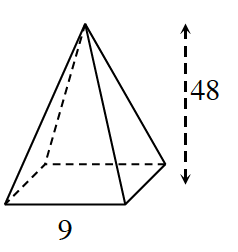### Home > CCG > Chapter 11 > Lesson 11.1.3 > Problem11-45

11-45.

Multiple Choice: The volume of the square-based pyramid with base edge $9$ units and height $48$ units is:

1. $324 \; \text{units}^3$

1. $1296 \; \text{units}^3$

1. $3888 \; \text{units}^3$

1. not enough informationRecall the volume of a pyramid is $\left(\frac{1}{3}\right)\left(\text{base area}\right)\left(\text{height}\right)$.

(b)